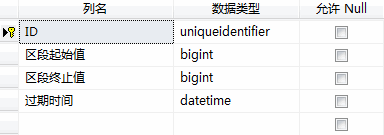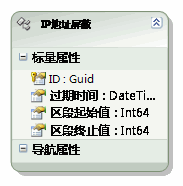IP地址的长度为32位，分为4段，每段8位，用十进制数字表示，每段数字范围为0255，段与段之间用句点隔开。”

TIP:

int取值范围：-2,147,483,648 到 2,147,483,647

uint取值范围：0 到 4,294,967,295

long取值范围：-9,223,372,036,854,775,808 到 9,223,372,036,854,775,807

127.0.0.1 -> 16777343

127.0.0.2 –> 33554559

/// <summary>

/// IP地址转为整数形式

/// </summary>

/// <returns>整数</returns>

public static long 转换为整数(this IPAddress ip)

{

int x = 3;

long o = 0;

{

o += (long)f << 8 * x--;

}

return o;

}

/// <summary>

/// 将整数转为IP地址

/// </summary>

/// <returns>IP地址</returns>

public static IPAddress 转换为IP地址(this long l)

{

var b = new byte;

for (int i = 0; i < 4; i++)

{

b[3 - i] = (byte)(l >> 8 * i & 255);

}

}

127.0.0.1 -> 2130706433

127.0.0.2 –> 2130706434

OK，确立了方案核心，下面开始设计SQLServer数据表：/// <summary>

/// 添加一个新的IP屏蔽区段

/// </summary>

/// <param name="IP区段起始值">起始IP，如61.51.200.0</param>

/// <param name="IP区段终止值">终止IP，如61.51.255.255</param>

/// <param name="过期时间">屏蔽截止时间</param>

/// <returns>ID</returns>

public static Guid 添加(string IP区段起始值, string IP区段终止值, DateTime 过期时间)

{

var id = Guid.NewGuid();

using (var c = new SiteMainEntities())

{

//检测是否已存在相同的IP屏蔽记录

var a = c.IP地址屏蔽.Where(f => f.区段起始值 == sip && f.区段终止值 == eip);

//如果存在则更新其过期时间

if (a.Count()>0)

{

var l = a.First();

if (l.过期时间 < 过期时间) l.过期时间 = 过期时间;

}

//不存在则正常添加一个新的屏蔽记录

else c.AddToIP地址屏蔽(new IP地址屏蔽 { ID = id, 过期时间 = 过期时间, 区段起始值 = sip, 区段终止值 = eip });

c.SaveChanges();

}

return id;

}

/// <summary>

/// 检测指定IP地址是否已受到屏蔽

/// </summary>

/// <param name="IP地址">要检测的IP地址</param>

/// <returns>是否属于已屏蔽的IP</returns>

public static bool 检测是否被屏蔽(string IP地址)

{

using (var c = new SiteMainEntities())

{

return c.IP地址屏蔽.Any(f => f.过期时间 > DateTime.Now && ip >= f.区段起始值 && ip <= f.区段终止值);

}

}

/// <summary>

/// 验证传入IP地址是否应被屏蔽。

/// </summary>

/// <param name="IP地址">待验证的IP</param>

/// <returns>是否应被屏蔽</returns>

{

var ip = IP地址.转换为整数();

return 过期时间 > DateTime.Now && this.区段起始值 <= ip && this.区段终止值 >= ip;

}

posted on 2008-11-05 05:58  斯克迪亚  阅读(8283)  评论(28编辑  收藏